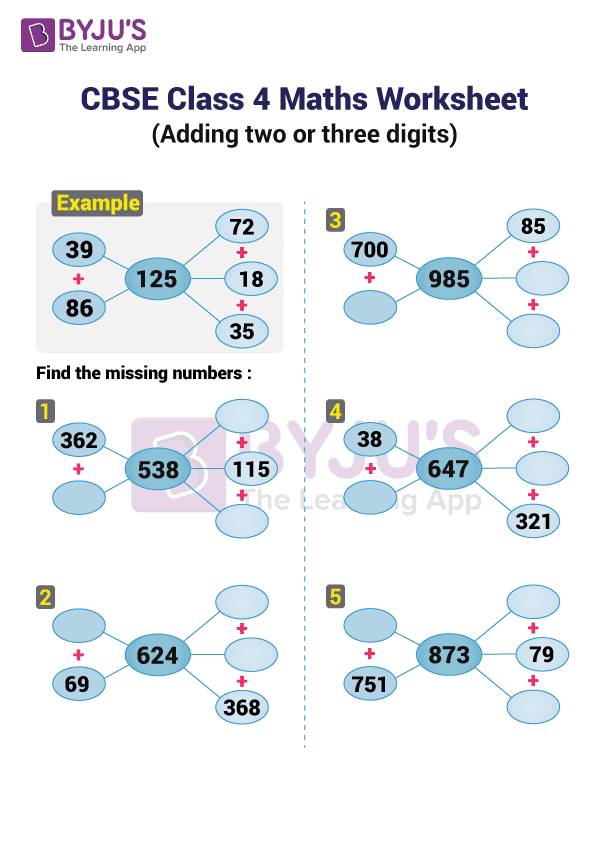# CBSE Class 4 Maths Worksheets

It is of utmost importance that class 4 students of CBSE board should practice the maths topics on daily basis which are taught in schools. For students practice, we are providing the CBSE Class 4 Maths Worksheets. These maths worksheets for class 4 can help the students to get a deeper understanding of the mathematics topics. The worksheets are designed as per the CBSE syllabus for class 4 and includes some very basics topics. CBSE class 4 maths practice worksheets pdf are engaging as it has been created by using graphics, diagrams for seeking students attention during learning.

Solving CBSE Class 4 Maths Worksheets will give students a more clarity on the topic and make their basics strong. They will see the practical aspect of the Maths topics and will learn its application simultaneously. Thus, their education will not be just a rote learning, instead it will add some value to their life.

The link below provide the CBSE Class 4 Maths Worksheets on different topics of Maths. Click on the respective links to download the CBSE Class 4 Maths Practice Worksheets PDF as mentioned below:

As the worksheets are for practice to students, therefore, we have not provided the answers. If students can not find the answer, they can ask their parents and search for a solution online. Moreover, they can ask their doubts by posting them in the comment section.

### Addition Worksheet for CBSE Class 4 Maths

Here in the picture below, we have explained the addition of 2 digit and 3 digit numbers through an example. We have also provided 5 questions on missing number based on the addition topic similar to the one explained through example.### Benefits of Solving of CBSE Class 4 Maths Worksheets

There are numerous benefits of solving the printed CBSE Maths Worksheet for Class 4. Here, we have listed a few of them.

1. These worksheet are curriculum specific and covers the all the important Maths topics.
2. Practising questions from these worksheets will engage students with the topics.
3. Worksheets will help students to learn the concepts taught in school in a concrete way.
4. Solving worksheets will improve the analytical and problem solving skills of students.
5. These worksheets will develop a regular schedule for studies.

Students can also solve CBSE sample paper for class 4 maths for further strengthening their basic understanding. Keep visiting BYJU’S website for other class-specific and subject-specific CBSE study materials for more efficiently exam preparation. Also, read GK Questions For Class 4.